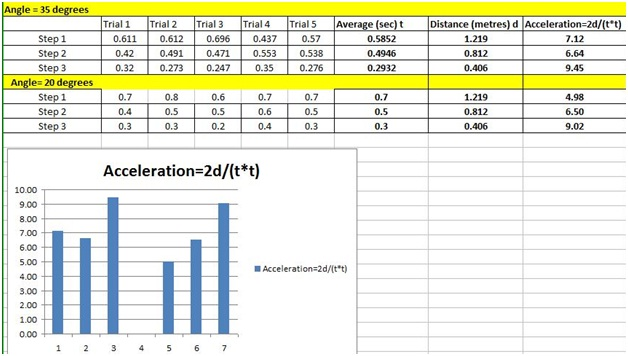Published on Mar 28, 2020

## Does Gravity Impact the Acceleration of a Rolling Object

### Abstract

The objective: To find out whether a golf ball's acceleration was impacted by gravity. I rolled it down a wooden plank, on a stack of books, timed it, then recorded it on Microsoft Excel.

### Research

#### How did the length of the molding affect the ball?

The speed increases with the increase of the length of the molding, however the acceleration stays relatively the same.

#### Was the acceleration exact for each interval?

No, the acceleration was not the same for each interval, because of inaccuracies in reading.

#### How did the angles impact on the speed of the golf ball?

As the angles increase, the speed of the golf ball increases. However the acceleration remains relatively the same.

#### Will the results vary with different lengths of molding?

Yes the results will vary, because it will be hard to time the exact moment the ball hits the ground with a small molding.

#### Will the acceleration be different with a different ball?

The acceleration is not supposed to change, however there are different conditions that affect the accuracy of the experiment.

#### How did the results change for the two times the project was done?

The 1st time the experiment was done the board that was used was short, so there were timing conditions that were less accurate. The 2nd time the experiment was done; the molding was long, causing the conditions to be better.

#### What is the formula to calculate acceleration?

The formula of acceleration is a = 2d / t2, (t=time, d=distance, a=acceleration).

#### What is acceleration?

Acceleration is a change in velocity.

#### What is the difference between acceleration and speed?

Acceleration is the rate of change of velocity with respect to time, and speed is velocity.

#### Did the acceleration change at each angle?

It is not supposed to change, but the acceleration did change at each angle, due to friction, timing, and the grooves of the golf ball.

### Purpose

To determine if there will be a change in acceleration of a golf ball due to gravity on Earth, when rolled down a wooden plank placed at various angles.

### Hypothesis

I think that the golf ball's speed will be greater when the angle becomes sharper. For example, if you stack a bunch of books on top of each other (angle= 175 degrees), compared to the same stack of books at a sharper angle (angle 100 degrees), the ball will travel faster on the second than the first.

### Materials

• Golf balls

• Stopwatch

• Microsoft excel

• Wooden plank

• Protractor

• Pen/pencil

• Ruler

• Books to stack

### Procedure

1. I stacked some books and set one side of molding on the books to create a ramp.

2. I used the protractor to measure the angle between the ramp and the floor. I adjusted the stack of books until I can got the ramp as close to 30 degrees as possible. Record the final angle in your notebook.

3. I used a ruler to mark 10 cm intervals along the ramp, starting at the floor and going upward.

4. I set the golf ball at a measured distance along the ramp. Then I timed how long it took for the golf ball to hit the floor after I let the ball go. I recorded both the distance I let the ball go and the time it took for the ball to travel the lengths along the ramp.

5. Repeat step four at different lengths along the ramp.

6. Graph your results. Put time on the x-axis, and distance traveled on the y axis.

7. Calculate the acceleration for the points you tested using the (a = 2d / r2 ) equation.

8. Try this experiment with different angles.

### Observation### Conclusion

In conclusion, the acceleration at each point was almost the same. The results varied because of improper timing and groove issues.